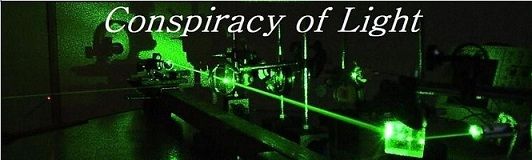The search for  new physics.

The mechanism of Time Dilation, and how it could be an illusion:

If one considers the classic thought experiment of time dilation, in which a light beam bounces between two mirrors travelling at relativistic speeds, it becomes clear that the time measured by a stationary observer vs. that of a moving observer is different if we rely on the time taken to move from one mirror to the other as the clock for both. From the perspective of the moving observer, the path is much longer, so it takes more time to reach the mirror. Thus time mathematically is directly inter-convertible with the velocity of light –if the velocity of the moving observer adds or subtracts from the speed of light, rather than being the same for both observers, then time dilation is reduced to a mechanical effect on clocks.

Particles have a wave nature, akin to that of light. These periodic vibrations pass to and fro across the body of the particle or around the periphery, at a velocity directly linked to the speed of light. Imagine a particle to be like a clock, in the shape of a disk, with a light path across the surface of the ring,  the light reflecting back and forth around the disk progressively through from 12 o’clock to 12 o’clock. This particle as a clock can be understood in exactly the same way as the Michelson Interferometer, and the explanation for the interferometer light speeds in the vertical and horizontal arms correspond to the transit of light across the segments of the disk shown in figure 3 below.

Michelson showed that if space contained a medium moving relative to the Earth, then the interferometer would experience a lengthening of the vertical (12 o’clock) path by 2D(1+ v2/2c2), and a lengthening of the 3 o’clock path by 2D(1+ v2/c2).

The 3 o’clock path would be longer by 2x the factor of the 12 o’clock path. However, since the clock in the path parallel to the velocity is altered by the Lorentz contraction by (1 - v2/c2)1/2, this path difference exactly cancels. Thus the measured speed of light appears to be the same for both the moving interferometer and the moving particle disk. However, the real path for light is longer by 1/(1 - v2/c2)1/2 all around the ring, and thus as a mechanical clock based on the speed of light, it will always run slower as it velocity increases with respect to any other inertial rest frame. However, absolute time does not actually change.

Fig. 3  The Particle as a Clock:This idea is consistent with the result of the Ives and Stillwell Experiment (1938, 1941). The moving atomic clock (protons) in the experiment emit a light ray which is frequency shifted by (1-v2/c2)1/2.

So if the Michelson interferometer is considered a light clock moving through space at some velocity v, then its time counting will slow with its velocity by (1-v2/c2)1/2. just like that predicted by Einstein, except it can be explained by a different reason - because the speed of light could be constant in some static frame of reference and because Lorentz's assumption that the physical Lorentz contraction is valid. This would mean that the passage of time can actually be absolute regardless of velocity, but that clocks based on the speed of light measured with respect to a preferred frame of reference will always read in error.
The perfection by which this process erases any detectability of our motion through space is truly astounding. If we consider the effect on the laser driving the Michelson interferometer, its constituents are also equally affected in the same way, lowering the frequency of the laser beam by the exact same amount. This means that not only is there no fringe shirt in the interferometer when it is moving, but an observer moving with it will always measure the propagation time along any arm to be exactly C . This is shown in our simulation of a Michelson interferometer here. Even the angles at which the light passes from one mirror to another is perfectly corrected during motion so that any observer moving with the device would only see the light paths moving rectilinearly, regarless of how fast they are moving. This was discussed in detail by Herbert Ives in his 1937 paper where he noted:

“The Fitzgerald-Lorentz contraction may be considered a means of introducing a tilt of the inclined mirror just sufficient to correct this error and bring the two emergent pulses into coincidence.”

This phenomena is discussed at greater length in a research article here. So we faced then with the possibilty that the speed of light is not actually constant for all inertial observers, and that real time does not actually slow down with velocity. Rather, this could simply be an illusion caused by our clocks being calibrated so to speak by the velocity of light with respect to absolute space. This is kind of like saying that we have a theromometer that is biased by changes in heat. It reads wrong due to the very thing we want to measure. So no matter what temperature it is, it alsways reads the same thing!References:

1) Ives, Herbert, “The Doppler Effect Considered in Relation to the Michelson-Morley Experiment.” J.O.S.A. Vol.27, 1937, p.389.

2) Ives, Herbert, “ An Experimental Study of the Rate of a Moving Atomic Clock.”  J.O.S.A. Vol. 28, 1938, P. 215.

3) Ives, Herbert, “ An Experimental Study of the Rate of a Moving Atomic Clock.” II J.O.S.A. Vol. 31, 1941, P. 369.